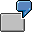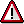Example: Threshold Value CheckThe logic of threshold value checks is explained in the following example.

100 bars of chocolate are produced. The standard price of a bar is USD 2. Single-level and multilevel price differences appear during production that are settled to the chocolate.

The threshold values amount to 20% for the periodic unit price variance and 20% for the multilevel variances.

The comparison price is the standard price.

Material Price Analysis

Multilevel Variances: Only the multilevel differences related to the quantity procured on a multilevel basis and the comparison price (in this case, the comparison price is the standard price) are included.

Percentage variance =

(Multilevel differences * 100%)/(Quantity procured on a multilevel basis * Standard price) = 16.67% (see 1)

Here, the threshold is not exceeded.

Variance of the periodic unit price: The recalculated price is compared with the comparison price (in this case, the standard price).

Percentage variance =

(Recalculated price - Standard price)*100%/Standard price = 25% (see 2)

Here, the threshold is exceeded.Because one of the two thresholds has been exceeded, the material remains faulty. You must either manually postprocess the errors or set an automatic error correction in the postprocessing options.